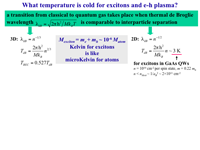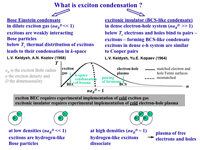## Introduction to quantum exciton gases and exciton condensation

### What is exciton condensation?An exciton is a bound pair of an electron and a hole. At low densities (naBD << 1, where aB is the exciton Bohr radius, n the density, and D the dimensionality), excitons are hydrogen-like Bose-particles. Because the exciton mass is small—even smaller than the free electron mass—exciton Bose-Einstein condensation (BEC) is expected to occur at relatively high temperatures, about 1 K, for experimentally accessible exciton densities. In a dense e-h system (naBD << 1), excitons are Cooper-pair-like Bose-particles and the exciton condensate, called the excitonic insulator, is analogous to the BCS superconductor state. In contrast to the BCS superconductor state, because the pairing in the excitonic insulator is due to electron-hole interaction, the pairs are neutral and the state is insulating. For the exciton condensation in a dense electron-hole system, nesting of the electron and hole Fermi-surfaces is required.

The nature of the exciton condensation is different in these two limits. In the case of BEC of excitons in the low density limit, excitons exist well above the critical temperature, the number of excitons is fixed and does not change at the critical temperature, and the critical temperature for the exciton condensation is determined by the statistical distribution in momentum space of weakly interacting bosons (i.e. excitons). In contrast, in the case of BCS-like condensation of excitons in the high density limit, excitons are formed at the critical temperature and the critical temperature for exciton condensation is determined by the pairing, similar to the case of Cooper pairs. The transition between the dilute and the dense limit is smooth and the condensation has a mixed nature for intermediate densities.

### How does one get a cold exciton gas?Cooling semiconductor lattice well below 1 K is routinely achieved in He refrigerators. Nevertheless, the exciton temperature can often considerably exceed that of the lattice. In fact, because the excitons can recombine, the exciton temperature is determined by the ratio of the exciton energy relaxation and recombination rates.

Because of the long lifetime and high cooling rate, the indirect excitons in CQWs form a system in which a cold exciton gas with temperatures below the critical temperature for exciton BEC can be created.

The long lifetimes of indirect excitons originate from the spatial separation between the electron and hole layers, resulting in a radiative lifetime of indirect excitons in CQWs samples that is more than three orders of magnitude longer than that of direct excitons in single QWs.

The cooling of hot photoexcited excitons down to the temperatures of the cold lattice, which occurs via emission of bulk LA phonons, is about three orders of magnitude faster for excitons in GaAs QWs than that in bulk GaAs. This is due to relaxation of the momentum conservation law in the direction perpendicular to the QW plane. Indeed, for quasi-2D systems the ground-state mode E = 0 couples to the continuum of the energy states E > E0 rather than to the single energy state E=E0=2Ms2 (s is the sound velocity) as it occurs in bulk semiconductors.